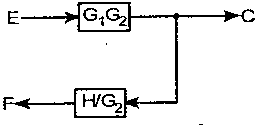# A power station supplies the peak load of 5o MW, 40 MW and 70 NIW to three localities. The annual load factor is 0.50 P.u. and the diversity factor of the load at the station is 1.55. The maximum demand on the station and average -load respectively will be

1.  120 MW, 60.8

2.  90 MW, 50.6

3.  103.2 MW, 51.61

4.   100 MW, 0.51

4

103.2 MW, 51.61

Explanation :
No Explanation available for this question

# Equivalent of the black diagram in the figure is

1.

2.

3.

4.

4Explanation :
No Explanation available for this question

# A generating station has a maximum demand of 50 MW, a load factor of 60%, a plant capacity factor of 45% and if the plant while running as per schedule, were fully loaded. The daily energy produced will

1.  400 MW

2.  720 MW

3.  500 MW

4.   600 MW

4

720 MW

Explanation :
No Explanation available for this question

# The transfer function of a linear system is

1.  ratio of the output, vo(t), and input vi(t)

2.  ratio of the derivatives of the output and the input

3.  ratio of the Laplace transform of the output and that of the input with all initial conditions zeros

4.  none of these

4

ratio of the Laplace transform of the output and that of the input with all initial conditions zeros

Explanation :
No Explanation available for this question

# The overall transfer function of the system in the given figure is

1.  G/1-GH

2.  2G/1- GH

3.  GH/1-GH

4.  2G/1-H

4

2G/1- GH

Explanation :
No Explanation available for this question

# A generating station has a maximum demand of 20 mW, load factor of 60%, a plant capacity factor of 50% and a plant use factor of 72%. What is the reserve capacity of the< plant, if the plant, while running as per schedule, were fully loaded

1.  10 MW

2.  15 MW

3.  2 MW

4.  5 MW

4

5 MW

Explanation :
No Explanation available for this question

# The transfer function of the system described by d2y/dt2+dy/dt=du/dt + 2u With u as input and. y as output is

1.  (s+2)/(s2+s)

2.  (s +1) /(s2 + s)

3.  s /(s2+s)

4.  2s/(s2+s)

4

(s+2)/(s2+s)

Explanation :
No Explanation available for this question

# In the above question, the maximum energy that could be produced daily is

1.  500 MWh/day

2.  360 MWh/day

3.  600 MWh/day

4.  720 MWh/day

4

500 MWh/day

Explanation :
No Explanation available for this question

# consider a feedback control system with loop transfer function G(s)H(s)=K(1+0.5s)/s(1+s)(1+2s) The type of the closed loop system is

1.  zero

2.  one

3.  two

4.  three

4

one

Explanation :
No Explanation available for this question

1.  s+1/(s+2)2

2.  2s+1/s2

3.  s+1/(s+1)2

4.  s+1/s2

4